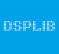My interest are Digital signal processing. Filter design. Fast algorithms of digital computing (fast Fourier transform, fast matrix computation) Adaptive filters Nonlinear filters My site is dsplib.org

## Re: Gaussian noise generation using non-pseudo random number generators

I think we must be very careful by using "true" random sources because theses things can be really random, but very difficult to make its realy white. Electrinic...

## Re: Gaussian noise generation using non-pseudo random number generators

Central random theorem is not good to Gaussian random generarion. Much better to use Box-Muller transformaton uniform pseudorandom. There are many uniform pseudorandom...

## Re: Off Topic: A geometry problem

We can just write AC+BC as function of C point.AC =sqrt(h^2+ (1-C)^2)BC = sqrt(h^2 + C^2)Here h is distance between line m and points A and B, C is C point coordinate...

Use this form to contact dsplib

Before you can contact a member of the *Related Sites:

• You must be logged in (register here)
• You must confirm you email address